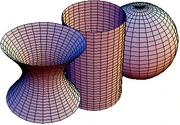11 Pages

Gaussian curvature is a part of differential geometry. It was discovered by Carl Friedrich Gauss.

## Explanation

There are three types of Gaussian curvature. The first type is negative curvature, which would be a hyperboloid. The second type is zero curvature which can be a cylinder. As you can see, there is a pattern, and thus positive curvature would be the opposite of a hyperboloid.Positive, negative and zero curvature.

There are two values involved in Gaussian curvature. These two values are both "curvatures"- κ1 and κ2. They are determined through the particular object. If one of these values is zero, then the Gaussian curvature is zero. However, if they are different, then the Gaussian curvature is negative. And if they are the same, the Gaussian curvature will be positive.

Community content is available under CC-BY-SA unless otherwise noted.